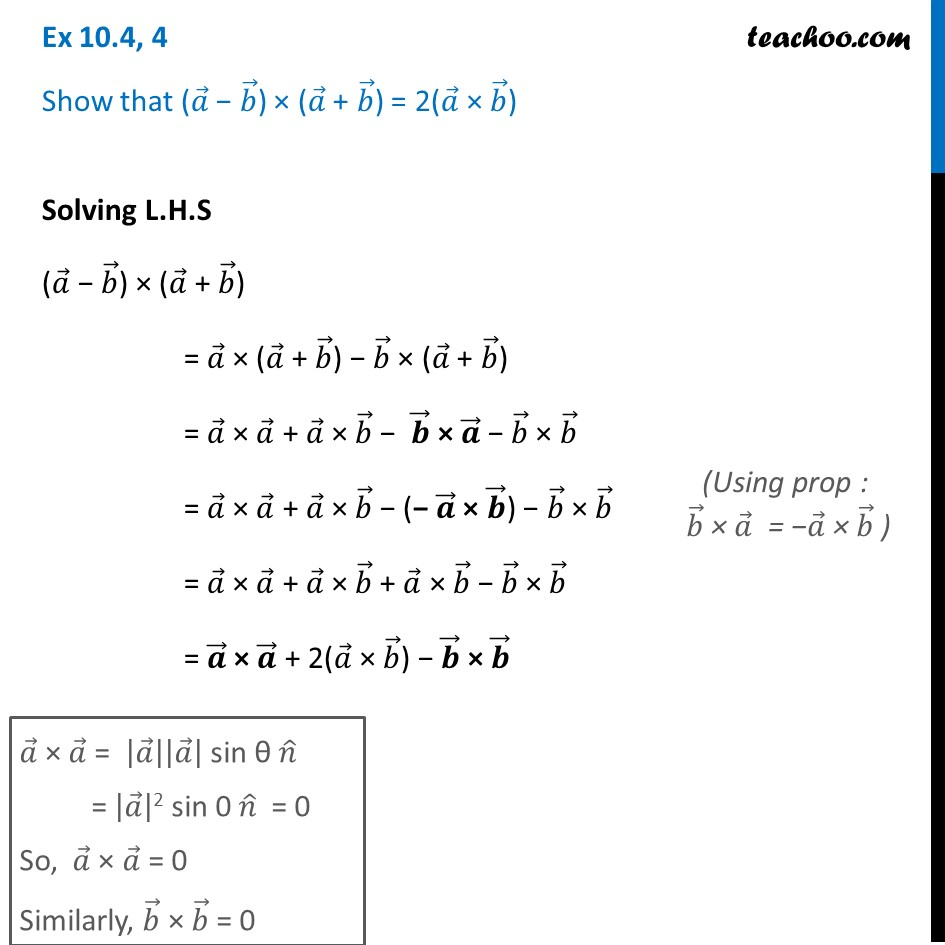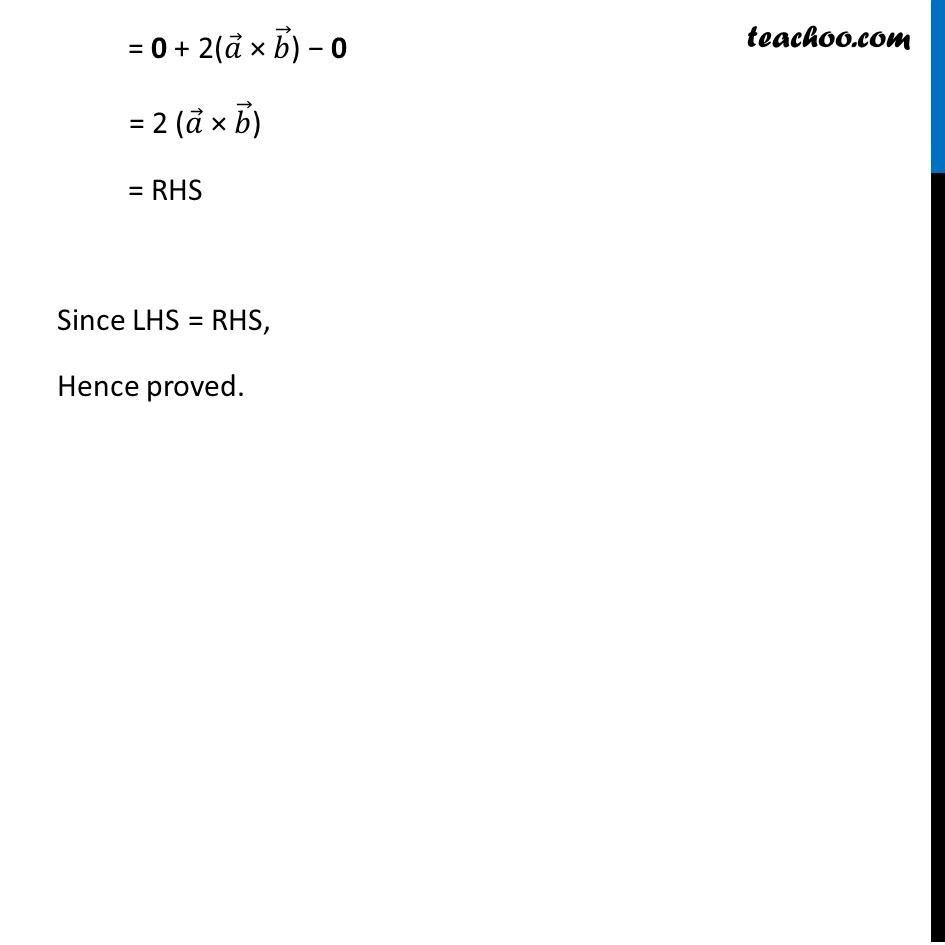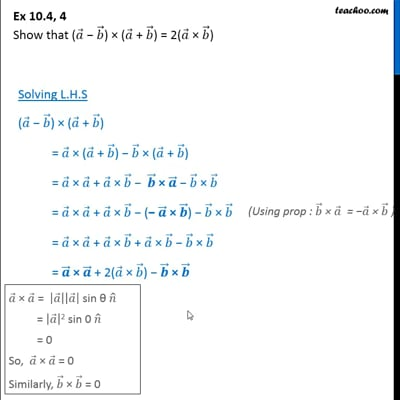Ex 10.4

Chapter 10 Class 12 Vector Algebra
Serial order wiseThis video is only available for Teachoo black users

Introducing your new favourite teacher - Teachoo Black, at only ₹83 per month

### Transcript

Ex 10.4, 4 Show that (𝑎 ⃗ − 𝑏 ⃗) × (𝑎 ⃗ + 𝑏 ⃗) = 2(𝑎 ⃗ × 𝑏 ⃗) Solving L.H.S (𝑎 ⃗ − 𝑏 ⃗) × (𝑎 ⃗ + 𝑏 ⃗) = 𝑎 ⃗ × (𝑎 ⃗ + 𝑏 ⃗) − 𝑏 ⃗ × (𝑎 ⃗ + 𝑏 ⃗) = 𝑎 ⃗ × 𝑎 ⃗ + 𝑎 ⃗ × 𝑏 ⃗ − 𝒃 ⃗ × 𝒂 ⃗ − 𝑏 ⃗ × 𝑏 ⃗ = 𝑎 ⃗ × 𝑎 ⃗ + 𝑎 ⃗ × 𝑏 ⃗ − (− 𝒂 ⃗ × 𝒃 ⃗) − 𝑏 ⃗ × 𝑏 ⃗ = 𝑎 ⃗ × 𝑎 ⃗ + 𝑎 ⃗ × 𝑏 ⃗ + 𝑎 ⃗ × 𝑏 ⃗ − 𝑏 ⃗ × 𝑏 ⃗ = 𝒂 ⃗ × 𝒂 ⃗ + 2(𝑎 ⃗ × 𝑏 ⃗) − 𝒃 ⃗ × 𝒃 ⃗ 𝑎 ⃗ × 𝑎 ⃗ = |𝑎 ⃗ ||𝑎 ⃗ | sin θ 𝑛 ̂ = |𝑎 ⃗ |2 sin 0 𝑛 ̂ = 0 So, 𝑎 ⃗ × 𝑎 ⃗ = 0 Similarly, 𝑏 ⃗ × 𝑏 ⃗ = 0 = 0 + 2(𝑎 ⃗ × 𝑏 ⃗) − 0 = 2 (𝑎 ⃗ × 𝑏 ⃗) = RHS Since LHS = RHS, Hence proved.